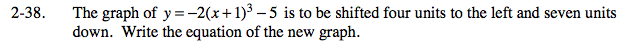### Home > PC > Chapter 2 > Lesson 2.2.1 > Problem2-38

2-38.

The graph of y = −2(x + 1)3 − 5 is to be shifted four units to the left and seven units down. Write the equation of the new graph. Homework Help ✎Write your equation for the new graph. Then use a graphing calculator to graph the original equation and your shifted equation. Check your work, identifying and correcting any mistakes.